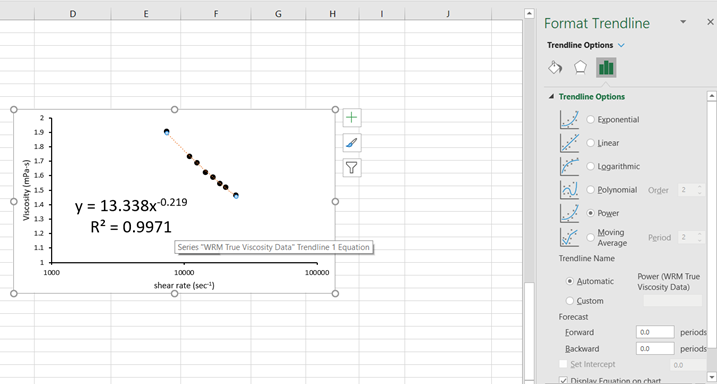# Application Note: Rheology Tools - Power Law Fluid Modeling

Knowing how to work with non-Newtonian fluids analytically is a vital skill across multiple fields. Scientists have been studying, analyzing, and characterizing non-Newtonian fluid behavior for decades and have developed analytical models to assist in their analysis. Mathematical model outputs can be used to interpolate data, rank and categorize fluids, and even help make calculations for fluid dynamics simulations. One such model used to characterize non-Newtonian fluids is the power law model.

The power law model is also known as the Ostwald de Waele relationship. It simply describes the behavior of a non-Newtonian fluid over the range of shear rates where the fit is performed. The power law model only has two fitting constants which is much simpler than other models that fit non-Newtonian fluids such as the Cross model or Carreau-Yasuda model which contain four and five fitting constants, respectively. In this application note, we will walk you through how to perform power law fitting on non-Newtonian fluids using Microsoft Excel.